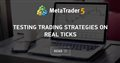# No error but code does not work.

im new to coding mlq5 and as a beginner i tried to make a EMA (exponantial moving avarage) bot that shows a BUY comment when the price was going under the 50 EMA line while the 50 EMA was above the 100 EMA and the 150 EMA.

even though there is no error in the code in backtesting it does not show the BUY comment when this signal is happening. i was hoping some of you could help me further.

```//+------------------------------------------------------------------+
//|                        Copyright 2020, MetaQuotes Software Corp. |
//|                                             https://www.mql5.com |
//+------------------------------------------------------------------+

int               green;
int               yellow;
int               red;

double            greenarray[],yellowarray[],redarray[];

MqlRates          PriceInformation[];

CPositionInfo     m_Position;

//+------------------------------------------------------------------+
//| Expert initialization function                                   |
//+------------------------------------------------------------------+
int OnInit()
{

ArraySetAsSeries (PriceInformation,true);
int Data=CopyRates(Symbol(),Period(),0,Bars(Symbol(),Period()),PriceInformation);

green  =iMA (_Symbol,_Period,50,0,MODE_EMA,PRICE_CLOSE);
yellow =iMA (_Symbol,_Period,100,0,MODE_EMA,PRICE_CLOSE);
red    =iMA (_Symbol,_Period,150,0,MODE_EMA,PRICE_CLOSE);

ArraySetAsSeries (greenarray,true);
ArraySetAsSeries (yellowarray,true);
ArraySetAsSeries (redarray,true);

CopyBuffer(green,0,0,3,greenarray);
CopyBuffer(yellow,0,0,3,yellowarray);
CopyBuffer(red,0,0,3,redarray);

return(INIT_SUCCEEDED);
}
//+------------------------------------------------------------------+
//| Expert deinitialization function                                 |
//+------------------------------------------------------------------+
void OnDeinit(const int reason)
{

}
//+------------------------------------------------------------------+
//| Expert tick function                                             |
//+------------------------------------------------------------------+
void OnTick()
{

if (greenarray>yellowarray>redarray
&& greenarray>PriceInformation.close)

{
}

}
//+------------------------------------------------------------------+
```Testing trading strategies on real ticks
• www.mql5.com
The article provides the results of testing a simple trading strategy in three modes: "1 minute OHLC" using only Open, High, Low and Close prices of minute bars; detailed modeling in "Every tick" mode, as well as the most accurate "Every tick based on real ticks" mode applying actual historical data. Comparing the results allows us to assess...

im new to coding mlq5 and as a beginner i tried to make a EMA (exponantial moving avarage) bot that shows a BUY comment when the price was going under the 50 EMA line while the 50 EMA was above the 100 EMA and the 150 EMA.

even though there is no error in the code in backtesting it does not show the BUY comment when this signal is happening. i was hoping some of you could help me further.

Have you tried printing out all values that are used in the if-statement at OnTick()? Comparing those values manually could explain why the "BUY" comment does not appear.

`  if (greenarray>yellowarray>redarray`
True = non-zero and false = zero so you get
 ```if( 3 < 2 < 1 ) if( false < 1 ) if(     0 < 1 ) if(     true  )``` ```if( 3 > 2 > 1 ) if(  true > 1 ) if(     1 > 1 ) if(     false ) ```

William Roeder:
True = non-zero and false = zero so you get

i'm not sure i follow how would this look in code?

William Roeder:
True = non-zero and false = zero so you get

This is a nice reply. Explains precisely what is happening.

So the code starts to compare an integer with a boolean due to it testing multiple conditions at the same time.

```void OnTick() {

double d_Green   = 4 ;
double d_Yellow  = 3 ;
double d_Red     = 2 ;
double d_Close   = 1 ;

string Message_1 = NULL ;
string Message_2 = NULL ;

// VARIANT 1
if ( d_Green > d_Yellow > d_Red
&& d_Green > d_Close          )
{
Message_1 = "VARIANT 1 IS TRUE " ;
}

// VARIANT 2
if ( ( d_Green > d_Yellow ) && ( d_Yellow > d_Red )
&& ( d_Green > d_Close  )     )
{
Message_2 = "VARIANT 2 IS TRUE " ;
}

Comment ( "DIVIDE AND RULE => " , Message_1 + Message_2 ) ;
}```

AIRAT SAFIN:
`if ( d_Green > d_Yellow > d_Red`
You can't do that; I already showed you why in #2

You are right that the logic of such a construction is incorrect
I did this to show the difference between right way and wrong way

William Roeder:
True = non-zero and false = zero so you get

thank you for your help this fixed my problem.

AIRAT SAFIN:
You are right that the logic of such a construction is incorrect
I did this to show the difference between right way and wrong way

I also wanted to thank you everybody in this thread helped me fix my problem :)

Reason: